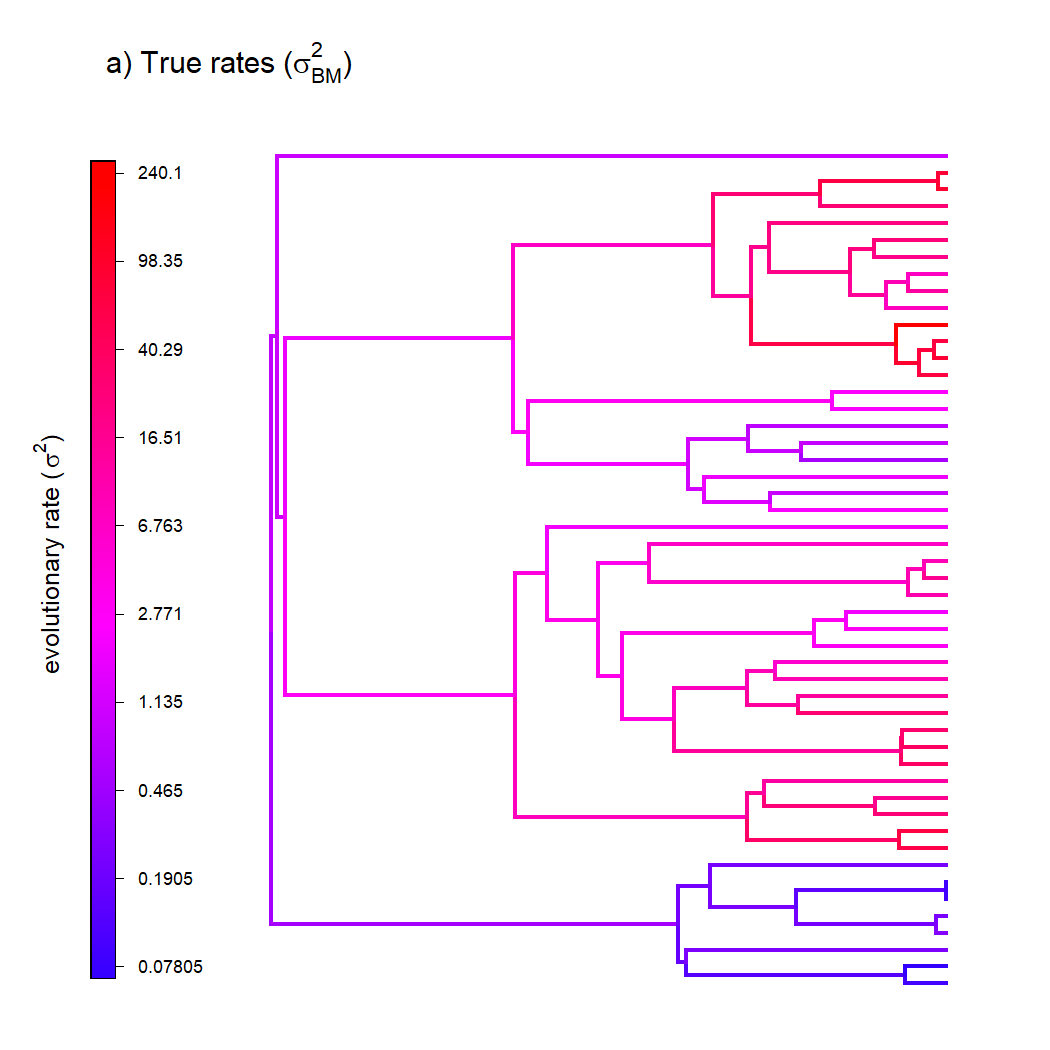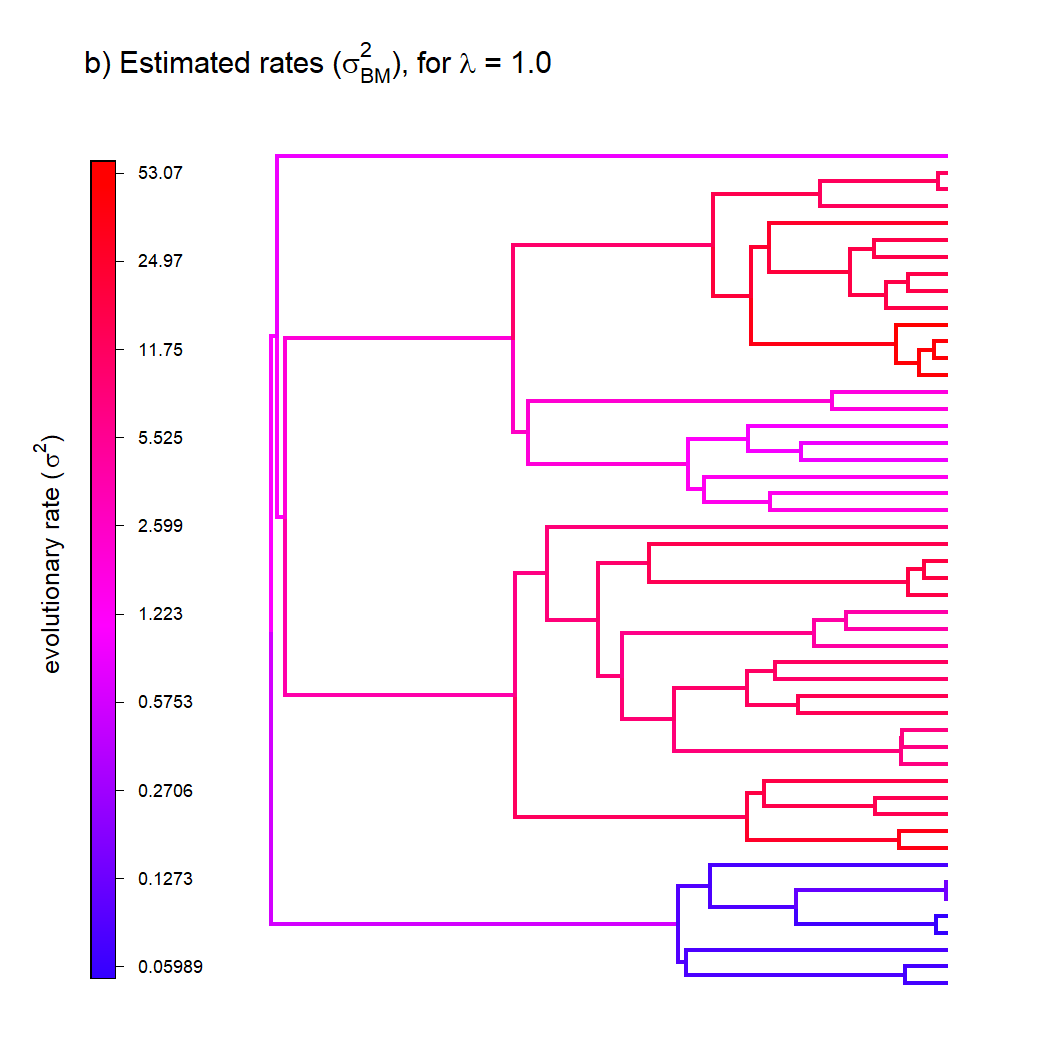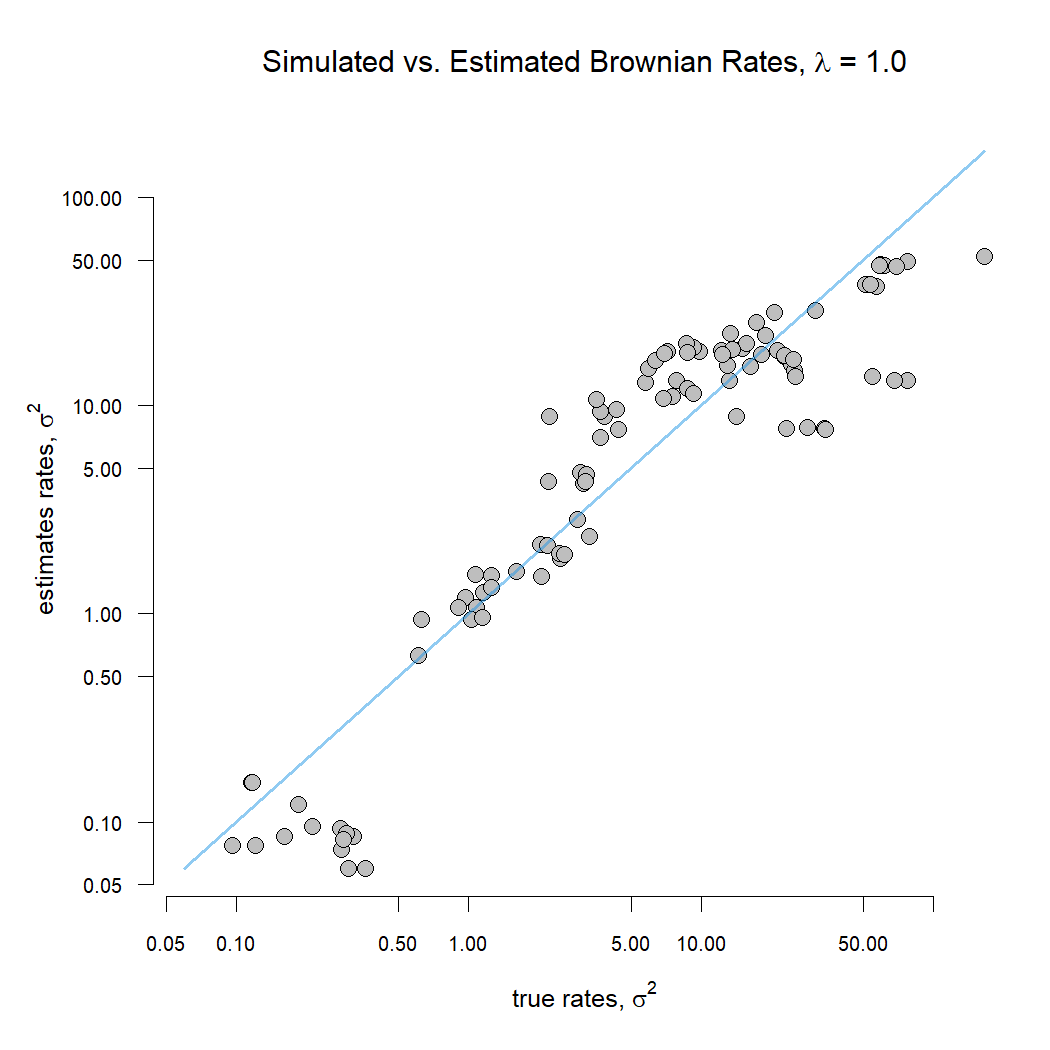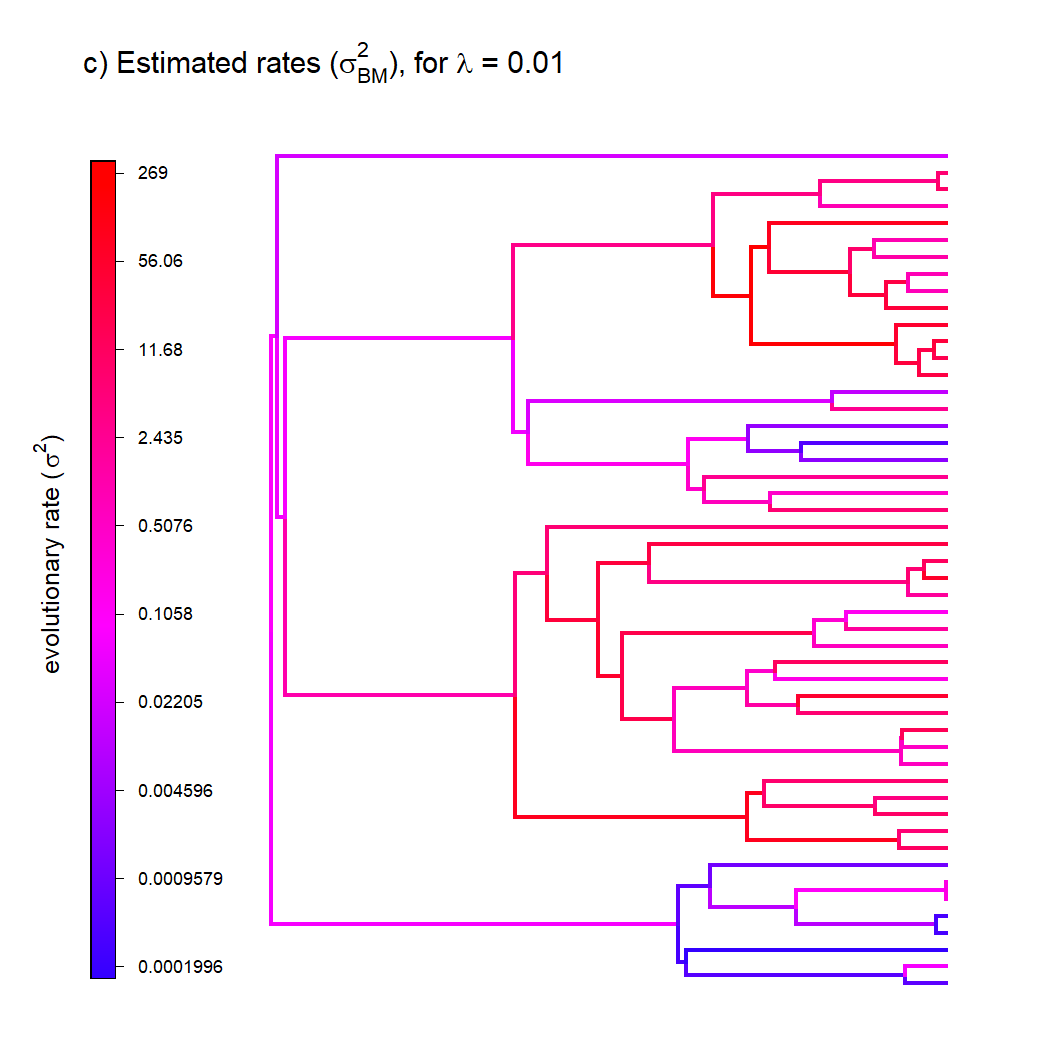## Tuesday, March 30, 2021

### Variable-rate Brownian motion evolution on a phylogeny: a penalized likelihood method in phytools

A few days ago, I shared a new variable rate Brownian motion evolution model for continuous traits on the tree.

In tests, the method performed pretty well, and I wrote a nice S3 `plot` method that helped a tweet about the method go 'viral' (or at least as viral as such things do).

Well, as it turns out things are a bit more complicated.

In particular, the model I was using actually ends up being non-identifiable if we expect to estimate both the Brownian motion rates of evolution (σi2) along all the edges of the tree, as well as the rate of Brownian evolution of the rate itself (let's call it σBM2).

What we can do very well, though, is estimate the rates under different scenarios for rate heterogeneity, if we assign a penalty term, λ, to the heterogeneity in rate among edges. This approach (of optimizing a problem using likelihood, but in which model complexity is penalized to an extent that's determined by the user) is called penalized likelihood, and it makes sense that it would work here because it is used in other rate-smoothing methods, including for phylogenetics. In this case, the penalty function that I'm using is the probability density of the rates σi2 on the tree. Not considering the data, this function will always have a maximum value with all rates equal!

A low value of the penalty term (say, λ = 0.01) accords very little penalty to rate variation among edges. On the other hand, a higher value (like, λ = 100) allows the rate to vary little. Intermediate λ (e.g., 1) gives “equal” weight to the probability of the data under the model, and the probability of the rate variation among edges, given a Brownian evolution process for rate variation.

Updated code for the function `multirateBM` is here.

Let's simulate some data and see how it works. In this case what I'm doing is simulating node states for the log-rate under Brownian motion, transforming the edges of my tree by the values at the subtending nodes, and then simulating data on the transformed tree.

``````library(phytools)
tree<-pbtree(n=50)
sig2<-exp(fastBM(tree,internal=TRUE))
tt<-tree
tt\$edge.length<-tt\$edge.length*apply(tt\$edge,1,
function(e,x) mean(x[e]),x=sig2)
x<-fastBM(tt)
``````

I'm actually going to “steal” the `plot` method for my `"multirateBM"` object class to visualize how this rate variation is distributed under on the tree in the generating model as follows:

``````object<-list()
class(object)<-"multirateBM"
object\$sig2<-sig2
object\$tree<-tree
plot(object,ftype="off",digits=4,mar=c(1.1,1.1,4.1,1.1))
title(main=expression(paste("a) True rates (",
``````Now let's fit our model – starting with a λ penalty coefficient of 1 – and see how the estimated rates compare.

``````fit.ml<-multirateBM(tree,x,lambda=1)
``````
``````## Beginning optimization....
## Optimization iteration 1. Using "Nelder-Mead" optimization method.
## Optimization iteration 2. Using "BFGS" optimization method.
## Done optimization.
``````
``````fit.ml
``````
``````## Multi-rate Brownian model using multirateBM.
##
## Fitted rates:
##       t35      t36       t4      t45      t46     t49     t50       t6
##  0.076963 0.077526 0.076633 0.059895 0.059905 0.15573 0.15573 0.075023 ....
##
## lambda penalty term: 1
## log-likelihood:  -104.205263
## AIC:  408.410527
##
## R thinks it has found a solution.
``````
``````plot(fit.ml,ftype="off",digits=4,mar=c(1.1,1.1,4.1,1.1))
title(main=expression(paste("b) Estimated rates (",
``````Since we know the true values of σ2 for each edge, we can easily check to see how well-correlated our estimated values are with our known, true rates:

``````options(scipen=999)
## generating sigma^2 by edge
SIG2<-apply(tree\$edge,1,function(e,x)
mean(x[e]),x=sig2)
## estimated sigma^2 by edge
est.SIG2<-apply(tree\$edge,1,function(e,x)
mean(x[e]),x=fit.ml\$sig2)
xylim<-range(c(SIG2,est.SIG2))
par(mar=c(5.1,5.1,4.1,1.1))
plot(SIG2,est.SIG2,pch=21,bg="grey",cex=1.5,bty="n",
xlab=expression(paste("true rates, ",sigma^2)),
ylab=expression(paste("estimates rates, ",sigma^2)),
xlim=xylim,ylim=xylim,log="xy",las=1,cex.axis=0.8)
lines(xylim,xylim,lwd=2,
col=make.transparent(palette(),0.5))
title(main=expression(paste(
"Simulated vs. Estimated Brownian Rates, ",
lambda," = 1.0")))
``````Now let's try again, but this time with λ = 0.01, to see what happens. We should expect our model to exhibit greater variation in the Brownian rate, σ2, among edges.

``````fit.ml2<-multirateBM(tree,x,lambda=0.01)
``````
``````## Beginning optimization....
## Optimization iteration 1. Using "Nelder-Mead" optimization method.
## Optimization iteration 2. Using "BFGS" optimization method.
## Done optimization.
``````
``````fit.ml2
``````
``````## Multi-rate Brownian model using multirateBM.
##
## Fitted rates:
##       t35     t36     t4      t45      t46     t49     t50       t6
##  0.000872 0.19456 0.0002 0.000589 0.000634 0.26076 0.26023 0.002561 ....
##
## lambda penalty term: 0.01
## log-likelihood:  -75.741123
## AIC:  351.482245
##
## R thinks it has found a solution.
``````
``````plot(fit.ml2,ftype="off",digits=4,mar=c(1.1,1.1,4.1,1.1))
title(main=expression(paste("c) Estimated rates (",
``````Indeed, this is exactly what we see as it's pretty clear that σ2 is changing much more abruptly from edge to edge in this plot compared to the one from before.

Lastly, we can do the same thing again – but this time with a much higher value of λ, say, 100,000. This should have the effect of eliminating variation in the Brownian rate among edges because of the very large penalty term we've created for rate variation.

Let's see:

``````fit.ml3<-multirateBM(tree,x,lambda=100000)
``````
``````## Beginning optimization....
## Optimization iteration 1. Using "Nelder-Mead" optimization method.
## Optimization iteration 2. Using "BFGS" optimization method.
## Done optimization.
``````
``````print(fit.ml3,printlen=Ntip(tree)+tree\$Nnode)
``````
``````## Multi-rate Brownian model using multirateBM.
##
## Fitted rates:
##    t35    t36     t4    t45    t46    t49    t50     t6    t30    t31    t26
## 16.505 16.505 16.505 16.505 16.505 16.505 16.505 16.505 16.505 16.505 16.505
##    t27     t8    t32    t33    t34    t14    t15    t12    t13    t18    t22
## 16.505 16.505 16.505 16.505 16.505 16.505 16.505 16.505 16.505 16.505 16.505
##    t23    t39    t41    t42     t3     t2    t10    t11     t5    t16    t17
## 16.505 16.505 16.505 16.505 16.505 16.505 16.505 16.505 16.505 16.505 16.505
##     t7    t20    t21    t40    t43    t44    t29    t28    t37    t38    t24
## 16.505 16.505 16.505 16.505 16.505 16.505 16.505 16.505 16.505 16.505 16.505
##    t25     t9    t19    t47    t48     t1     51     52     53     54     55
## 16.505 16.505 16.505 16.505 16.505 16.505 16.505 16.505 16.505 16.505 16.505
##     56     57     58     59     60     61     62     63     64     65     66
## 16.505 16.505 16.505 16.505 16.505 16.505 16.505 16.505 16.505 16.505 16.505
##     67     68     69     70     71     72     73     74     75     76     77
## 16.505 16.505 16.505 16.505 16.505 16.505 16.505 16.505 16.505 16.505 16.505
##     78     79     80     81     82     83     84     85     86     87     88
## 16.505 16.505 16.505 16.505 16.505 16.505 16.505 16.505 16.505 16.505 16.505
##     89     90     91     92     93     94     95     96     97     98     99
## 16.505 16.505 16.505 16.505 16.505 16.505 16.505 16.505 16.505 16.505 16.505
##
## lambda penalty term: 100000
## log-likelihood:  -136.439244
## AIC:  472.878488
##
## R thinks it has found a solution.
``````

Indeed all the rates are now more or less the same, and (not coincidentally) they correspond to our ML rate under a constant-rate Brownian model, just as you'd expect.

``````geiger::fitContinuous(tree,x)
``````
``````## GEIGER-fitted comparative model of continuous data
##  fitted 'BM' model parameters:
##  sigsq = 16.505384
##  z0 = -1.119807
##
##  model summary:
##  log-likelihood = -136.439253
##  AIC = 276.878506
##  AICc = 277.133825
##  free parameters = 2
##
## Convergence diagnostics:
##  optimization iterations = 100
##  failed iterations = 0
##  number of iterations with same best fit = 100
##  frequency of best fit = 1.00
##
##  object summary:
##  'lik' -- likelihood function
##  'bnd' -- bounds for likelihood search
##  'res' -- optimization iteration summary
##  'opt' -- maximum likelihood parameter estimates
``````

Neat.

I think it's probably fairly obvious that if λ of the penalty term is low, then the rates can just take any value that they like; if λ is high, then they're all the same; and if λ is just right (i.e., somewhere in the middle) then the rates, σ2, will evolve according to a Brownian process. What value of λ this will be, though, should depend on the scale of our data and rates!